# How to correctly set a diagonal or non-X, Y fields with maximum field value

• 0 replies
• 331 views

Userlevel 6+2
• Zemax Staff
• 108 replies

Sometimes we want to set a diagonal field point with same radial amount same as those X or Y field points. However, if it's not correctly set up, we may find the optical performance for these diagonal points are slightly different compared to those X or Y field points.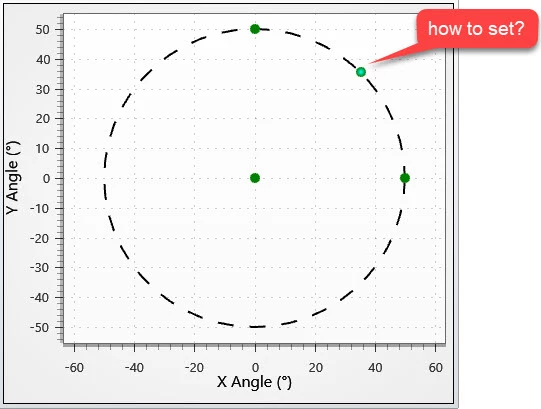Here let's look at an example how to correctly set up a diagonal field point.

First, we open the built-in file \Documents\Zemax\Samples\Sequential\Objectives\Wide angle lens 100 degree field.zmx

This system has a maximum field angle = 50 degrees.

Let's say we want to set a diagonal field (angle_x, angle_y), where the angle_x = angle_y and we need to find the value of angle_x/y.

Based on Help file, we know the Field Angle is corresponded to direction cosines LMN in following equations.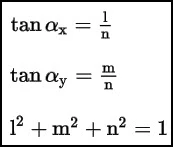If we want to build a diagonal field with same radial field amount as the field point (50, 0) or (50, 0), we need to make sure they have same value of L^2 + M^2. Therefore, we know the L and M for the diagonal value can calculated as:

L = M = sqrt(sin(50)^2 / 2) = 0.541675

And N = sqrt(1-L^2-M^2) = 0.642788

Then we have

angle_x = angle_y = atan(L/N) = atan(M/N) = 40.12074

Now we can type it in Field Data Editor as below.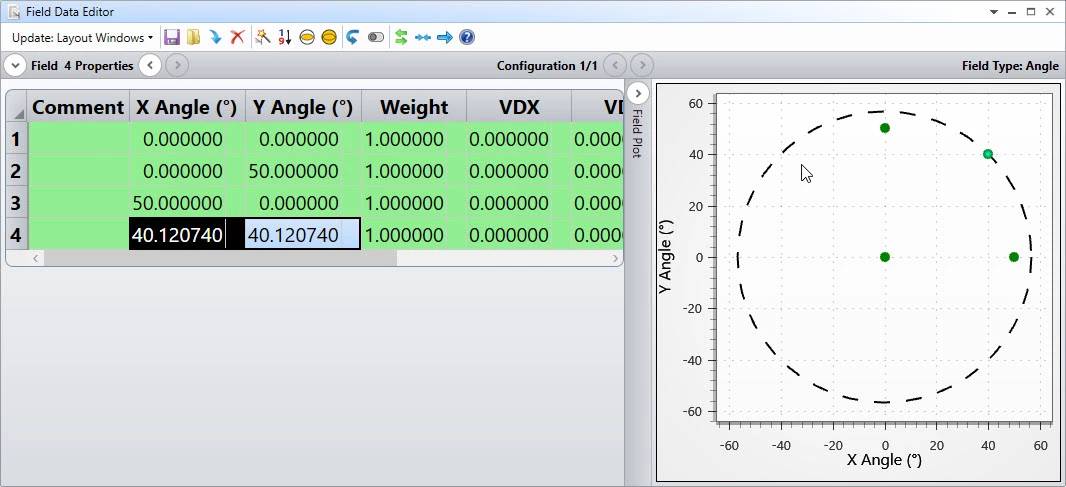Let's check whether Field 4 has same performance as Field 2 and 3.

First we use REAR in the merit function. As shown below, the radial image coordinate of all three fields are exactly same.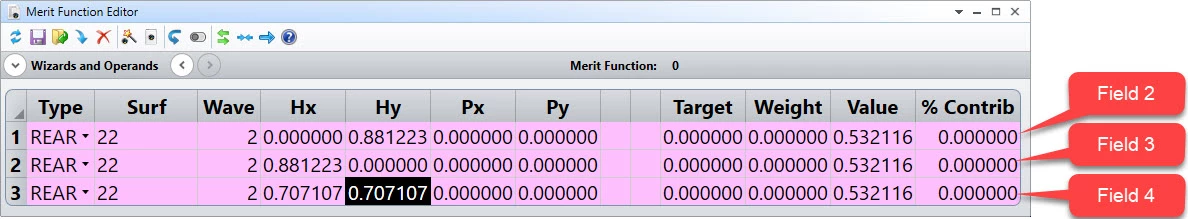Here I use a trick to get the correct (Hx, Hy) for the desire field by using Single Ray Trace tool as below.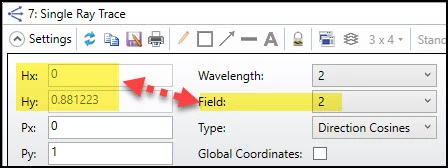To compare the value in Spot Diagram or MTF, we first set the TAN value for the diagonal field to 45 degrees.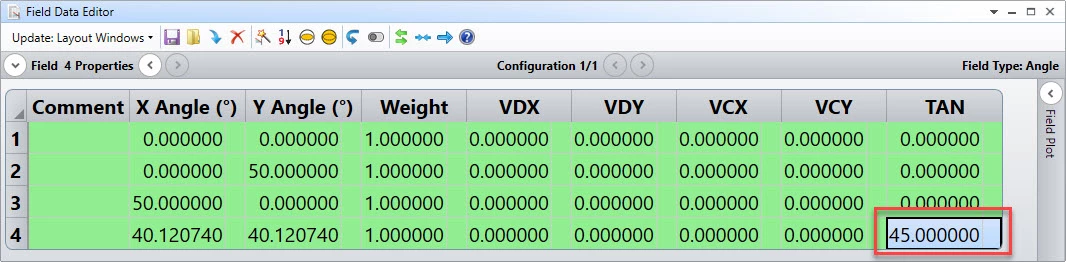It can be seen the result match perfectly in Spot Diagram and MTF.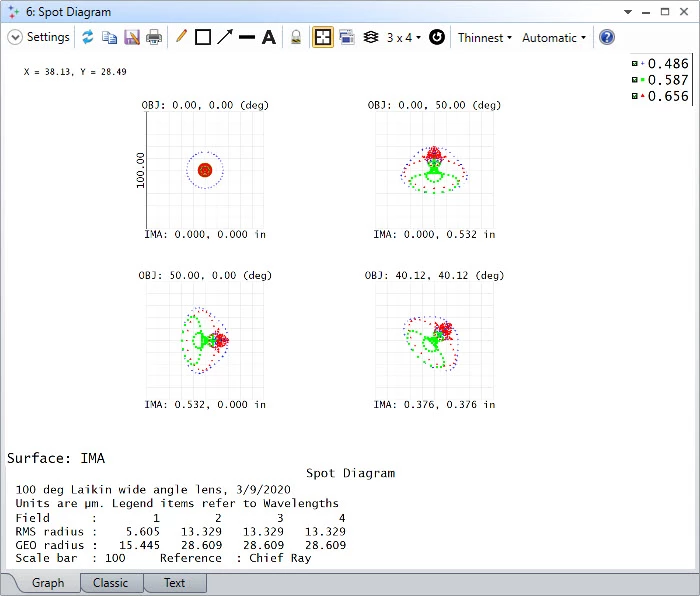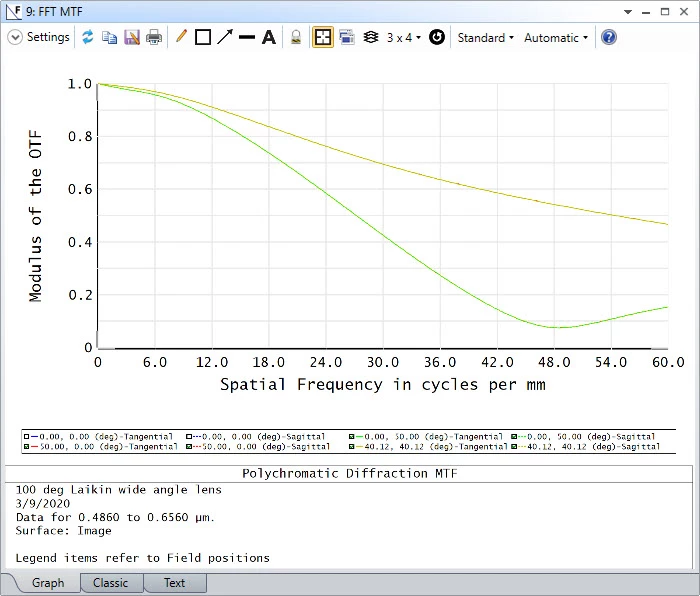For the meaning of TAN in Field Data Editor, it's suggested to read the following knowledge base article.

Understanding tangential/sagittal in OpticStudio and how to rotate rays